JMSLTM Numerical Library 7.2.0
com.imsl.math

## Class BoundedVariableLeastSquares

• All Implemented Interfaces:
Serializable, Cloneable

```public class BoundedVariableLeastSquares
extends Object
implements Serializable, Cloneable```
Solve a linear least-squares problem with bounds on the variables. `BoundedVariableLeastSquares` solves the least-squares problem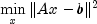subject to the conditions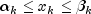for all k.

This algorithm is a generalization of `NonNegativeLeastSquares`, that solves the least-squares problem, Ax = b, subject to all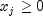. NonNegativeLeastSquares is based on the subroutine NNLS which appeared in Lawson and Hanson (1974). The additional work on bounded variable least squares was published in a later reprint (Lawson and Hanson, 1995).

`NonNegativeLeastSquares`, Example, Serialized Form
• ### Nested Class Summary

Nested Classes
Modifier and Type Class and Description
`static class ` `BoundedVariableLeastSquares.TooManyIterException`
Maximum number of iterations exceeded.
• ### Constructor Summary

Constructors
Constructor and Description
```BoundedVariableLeastSquares(double[][] a, double[] b, double[] lowerBound, double[] upperBound)```
Construct a new BoundedVariableLeastSquares instance to solve Ax-b subject to bounds on the variables.
• ### Method Summary

Methods
Modifier and Type Method and Description
`double[]` `getDualSolution()`
Returns the dual solution vector, w.
`int` `getIterations()`
Returns the number of iterations used to find the solution.
`double` `getResidualNorm()`
Returns the euclidean norm of the residual vector,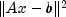.
`double[]` `getSolution()`
Returns the solution to the problem.
`void` `setMaxIterations(int maxIterations)`
Sets the maximum number of iterations.
`void` `setTolerance(double tolerance)`
Sets the internal tolerance used to determine the relative linear dependence of a column vector for a variable moved from its initial value.
`void` `solve()`
Find the solution x to the problem for the current constraints.
• ### Methods inherited from class java.lang.Object

`clone, equals, finalize, getClass, hashCode, notify, notifyAll, toString, wait, wait, wait`
• ### Constructor Detail

• #### BoundedVariableLeastSquares

```public BoundedVariableLeastSquares(double[][] a,
double[] b,
double[] lowerBound,
double[] upperBound)```
Construct a new BoundedVariableLeastSquares instance to solve Ax-b subject to bounds on the variables. Each upper bound must be greater than or equal to the corresponding lower bound.
Parameters:
`a` - the `double` input matrix.
`b` - a `double` array of length `a.length`.
`lowerBound` - a `double` array of length n containing lower bounds. Use `Double.NEGATIVE_INFINITY` for variables which are not bounded below.
`upperBound` - a `double` array of length n containing upper bounds. Use `Double.POSITIVE_INFINITY` for variables which are not bounded above.
• ### Method Detail

• #### getDualSolution

`public double[] getDualSolution()`
Returns the dual solution vector, w. If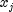is at neither its upper nor lower bound then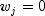. Ifis at its lower bound then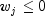. Ifis at its upper bound then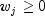. If the upper and lower bound for the j -th variable are equal, fixing the value of, then the value of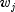is arbitrary.
Returns:
a `double` array containing the dual solution vector, w.
• #### getIterations

`public int getIterations()`
Returns the number of iterations used to find the solution.
Returns:
an `int` containing the number of iterations.
• #### getResidualNorm

`public double getResidualNorm()`
Returns the euclidean norm of the residual vector,.
Returns:
a `double` containing the euclidean norm of the residual vector.
• #### getSolution

`public double[] getSolution()`
Returns the solution to the problem.
Returns:
a `double` array containing the solution.
• #### setMaxIterations

`public void setMaxIterations(int maxIterations)`
Sets the maximum number of iterations.
Parameters:
`maxIterations` - an `int` specifying the maximum number of iterations. The default is three times ``` a.length```.
• #### setTolerance

`public void setTolerance(double tolerance)`
Sets the internal tolerance used to determine the relative linear dependence of a column vector for a variable moved from its initial value.
Parameters:
`tolerance` - a `double` value specifying the tolerance. The default value is 1.0e-7.
• #### solve

```public void solve()
throws BoundedVariableLeastSquares.TooManyIterException```
Find the solution x to the problem for the current constraints.
Throws:
`BoundedVariableLeastSquares.TooManyIterException`
JMSLTM Numerical Library 7.2.0